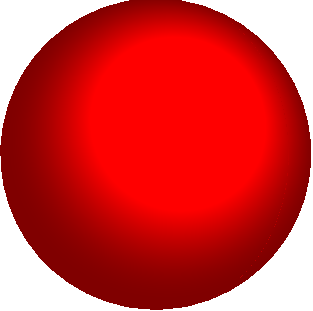Appendix E

Miscellaneous derivations

##### E.1. Relation between the areas of the faces of a tetrahedronFor the tetrahedron shown, we prove that:

$\frac{d{A}_{1}}{d{A}_{\left(n\right)}}={n}_{1}\text{\hspace{0.17em}}\text{\hspace{0.17em}}\text{\hspace{0.17em}}\text{\hspace{0.17em}}\text{\hspace{0.17em}}\text{\hspace{0.17em}}\text{\hspace{0.17em}}\text{\hspace{0.17em}}\text{\hspace{0.17em}}\text{\hspace{0.17em}}\frac{d{A}_{2}}{d{A}_{\left(n\right)}}={n}_{2}\text{\hspace{0.17em}}\text{\hspace{0.17em}}\text{\hspace{0.17em}}\text{\hspace{0.17em}}\text{\hspace{0.17em}}\text{\hspace{0.17em}}\text{\hspace{0.17em}}\text{\hspace{0.17em}}\text{\hspace{0.17em}}\frac{d{A}_{3}}{d{A}_{\left(n\right)}}={n}_{3}\text{\hspace{0.17em}}\text{\hspace{0.17em}}\text{\hspace{0.17em}}\text{\hspace{0.17em}}\text{\hspace{0.17em}}\text{\hspace{0.17em}}\text{\hspace{0.17em}}$

where $d{A}_{\left(n\right)}$ is the area of the face with normal n, and $d{A}_{i}$ is the face with normal $-{e}_{i}$

Note that

$d{A}_{1}=\frac{1}{2}bc\text{\hspace{0.17em}}\text{\hspace{0.17em}}\text{\hspace{0.17em}}\text{\hspace{0.17em}}\text{\hspace{0.17em}}\text{\hspace{0.17em}}\text{\hspace{0.17em}}\text{\hspace{0.17em}}\text{\hspace{0.17em}}\text{\hspace{0.17em}}\text{\hspace{0.17em}}d{A}_{2}=\frac{1}{2}ac\text{\hspace{0.17em}}\text{\hspace{0.17em}}\text{\hspace{0.17em}}\text{\hspace{0.17em}}\text{\hspace{0.17em}}\text{\hspace{0.17em}}\text{\hspace{0.17em}}\text{\hspace{0.17em}}\text{\hspace{0.17em}}\text{\hspace{0.17em}}d{A}_{3}=\frac{1}{2}ab$

Note also that we can compute the area of the face with normal n by taking cross products of the vectors defining the sides of the face:

$\begin{array}{l}d{A}_{n}n=\frac{1}{2}\left(c{e}_{3}-b{e}_{2}\right)×\left(a{e}_{1}-b{e}_{2}\right)=\frac{1}{2}\left(bc{e}_{1}+ac{e}_{2}+ab{e}_{3}\right)\\ ⇒d{A}_{n}n=d{A}_{1}{e}_{1}+d{A}_{2}{e}_{2}+d{A}_{3}{e}_{3}\\ ⇒n=\frac{d{A}_{1}}{d{A}_{n}}{e}_{1}+\frac{d{A}_{2}}{d{A}_{n}}{e}_{2}+\frac{d{A}_{3}}{d{A}_{n}}{e}_{3}\end{array}$

so that

$\frac{d{A}_{1}}{d{A}_{\left(n\right)}}={n}_{1}\text{\hspace{0.17em}}\text{\hspace{0.17em}}\text{\hspace{0.17em}}\text{\hspace{0.17em}}\text{\hspace{0.17em}}\text{\hspace{0.17em}}\text{\hspace{0.17em}}\text{\hspace{0.17em}}\text{\hspace{0.17em}}\text{\hspace{0.17em}}\frac{d{A}_{2}}{d{A}_{\left(n\right)}}={n}_{2}\text{\hspace{0.17em}}\text{\hspace{0.17em}}\text{\hspace{0.17em}}\text{\hspace{0.17em}}\text{\hspace{0.17em}}\text{\hspace{0.17em}}\text{\hspace{0.17em}}\text{\hspace{0.17em}}\text{\hspace{0.17em}}\frac{d{A}_{3}}{d{A}_{\left(n\right)}}={n}_{3}\text{\hspace{0.17em}}$

as required.

E.2. Relation between area elements before and after deformationConsider an element of area $d{A}_{0}$ with normal ${n}^{0}$ in a deformable solid.  Suppose the solid is deformed, and let ${F}_{ij}$ denote the components of the deformation gradient tensor. The area element deforms with the solid, and has a new area $dA$ and normal n.  We plan to prove that the deformed area element is related to its undeformed area through

$dA{n}_{i}^{}=J{F}_{ki}^{-1}{n}_{k}^{0}d{A}_{0}$

where $J=\mathrm{det}\left({F}_{ij}\right)$

Start by noting that the area before deformation can be computed by taking the cross product of two infinitesimal vectors $d{v}_{i},\text{\hspace{0.17em}}\text{\hspace{0.17em}}\text{\hspace{0.17em}}d{w}_{i}$ bounding the area element in the undeformed configuration

$d{A}_{0}{n}_{i}^{0}={\in }_{ijk}d{v}_{j}d{w}_{k}$

Note that the infinitesimal vectors map to ${F}_{ij}d{w}_{j}$, ${F}_{ij}d{v}_{j}$ in the deformed configuration.  Therefore

$dA{n}_{i}^{}={\in }_{ijk}{F}_{jm}d{v}_{m}{F}_{kn}d{w}_{n}$

Let ${F}_{ij}^{-1}$ denote the inverse of the deformation gradient tensor, i.e. ${F}_{ik}{F}_{kj}^{-1}={\delta }_{ij}$. Then, we could write

$dA{n}_{i}^{}={F}_{pl}{F}_{li}^{-1}{\in }_{pjk}{F}_{jm}{F}_{kn}d{v}_{m}d{w}_{n}$

Now, recall the identity

$\begin{array}{l}\lambda =\mathrm{det}A\text{\hspace{0.17em}}\text{\hspace{0.17em}}\text{\hspace{0.17em}}\text{\hspace{0.17em}}\text{\hspace{0.17em}}\text{\hspace{0.17em}}\text{\hspace{0.17em}}\text{\hspace{0.17em}}\text{\hspace{0.17em}}\equiv \text{\hspace{0.17em}}\text{\hspace{0.17em}}\text{\hspace{0.17em}}\text{\hspace{0.17em}}\text{\hspace{0.17em}}\lambda =\text{\hspace{0.17em}}\text{\hspace{0.17em}}\frac{1}{6}{\in }_{ijk}{\in }_{lmn}{A}_{li}{A}_{mj}{A}_{nk}\text{\hspace{0.17em}}\\ \text{\hspace{0.17em}}\text{\hspace{0.17em}}\text{\hspace{0.17em}}\text{\hspace{0.17em}}\text{\hspace{0.17em}}\text{\hspace{0.17em}}\text{\hspace{0.17em}}\text{\hspace{0.17em}}\text{\hspace{0.17em}}\text{\hspace{0.17em}}\text{\hspace{0.17em}}\text{\hspace{0.17em}}\text{\hspace{0.17em}}\text{\hspace{0.17em}}\text{\hspace{0.17em}}\text{\hspace{0.17em}}\text{\hspace{0.17em}}\text{\hspace{0.17em}}\text{\hspace{0.17em}}\text{\hspace{0.17em}}\text{\hspace{0.17em}}\text{\hspace{0.17em}}\text{\hspace{0.17em}}\text{\hspace{0.17em}}\text{\hspace{0.17em}}\text{\hspace{0.17em}}\text{\hspace{0.17em}}\text{\hspace{0.17em}}\text{\hspace{0.17em}}\text{\hspace{0.17em}}\text{\hspace{0.17em}}⇔\text{\hspace{0.17em}}\text{\hspace{0.17em}}\text{\hspace{0.17em}}{\in }_{lmn}\lambda \text{\hspace{0.17em}}\text{\hspace{0.17em}}=\text{\hspace{0.17em}}\text{\hspace{0.17em}}\text{\hspace{0.17em}}{\in }_{ijk}{A}_{il}{A}_{jm}{A}_{kn}\end{array}$

so that

$dA{n}_{i}^{}={F}_{li}^{-1}J{\in }_{lmn}d{v}_{m}d{w}_{n}={F}_{li}^{-1}Jd{A}_{\text{\hspace{0.17em}}0}{n}_{l}^{0}$

where $J=\mathrm{det}\left({F}_{ij}\right)$, giving the required result.

E.3. Time derivatives of integrals over volumes within a deforming solidConsider a deformable solid.  Let ${\rho }_{0}$ denote the mass density of the solid in the original configuration, and let $\rho$ denote the mass density in the deformed configuration. (Both ${\rho }_{0}$ and $\rho$ can vary with position in the solid). Let  ${V}_{0}$ denote a closed region within the undeformed solid, and let V be the same region of the solid in the deformed configuration.  Suppose that

${v}_{i}=\frac{\partial {u}_{i}}{\partial t}$

denotes the velocity field within the body.  We shall show that

$\frac{d}{dt}\left\{\underset{V}{\int }\rho \text{\hspace{0.17em}}{v}_{i}dV\right\}=\underset{V}{\int }\rho \frac{d{v}_{i}}{dt}dV$

and also that

$\frac{d}{dt}\left\{\underset{V}{\int }{\in }_{ijk}{y}_{j}\rho \text{\hspace{0.17em}}{v}_{k}dV\right\}=\underset{V}{\int }\rho {\in }_{ijk}{y}_{j}\frac{d{v}_{k}}{dt}dV\text{\hspace{0.17em}}$

At first glance, this looks obvious $–$ just take the derivative under the integral sign.  You can’t do this, however, because the volume V changes with time, as the solid is deforming.  In addition, the mass density varies with time, because of the deformation, so even if we could take the time derivative under the integral, we’d end up with an additional term. To do the derivative properly, we first need to change variables so the integral is evaluated over the undeformed volume (which is independent of time). Thus

$\underset{V}{\int }\rho {v}_{i}dV=\underset{{V}_{0}}{\int }\rho {v}_{i}Jd{V}_{0}=\underset{{V}_{0}}{\int }{\rho }_{0}{v}_{i}d{V}_{0}$

where

$J=\mathrm{det}\left({F}_{ij}\right)=\frac{dV}{d{V}_{0}}$

and we have recalled a result from the Kinematics section

$J\rho ={\rho }_{0}$

Now, we can happily differentiate.  The mass density in the undeformed configuration does not vary with time, so that

$\frac{d}{dt}\left\{\underset{{V}_{0}}{\int }{\rho }_{0}\text{\hspace{0.17em}}{v}_{i}d{V}_{0}\right\}=\underset{{V}_{0}}{\int }{\rho }_{0}\frac{d{v}_{i}}{dt}d{V}_{0}=\underset{V}{\int }\rho \frac{d{v}_{i}}{dt}dV$

The last expression was obtained by changing variables in the integral back to the deformed configuration.  This is the first result we wanted.

To show the second result, follow exactly the same procedure, until you obtain

$\frac{d}{dt}\left\{\underset{{V}_{0}}{\int }{\in }_{ijk}{y}_{j}\text{\hspace{0.17em}}{v}_{k}{\rho }_{0}\text{\hspace{0.17em}}d{V}_{0}\right\}=\underset{{V}_{0}}{\int }{\rho }_{0}{\in }_{ijk}\left(\frac{d{y}_{j}}{dt}{v}_{k}+{y}_{j}\frac{d{v}_{k}}{dt}\right)d{V}_{0}$

Now, observe that

${\in }_{ijk}\frac{d{y}_{j}}{dt}{v}_{k}={\in }_{ijk}{v}_{j}{v}_{k}=0$

(the cross product of two parallel vectors is zero) so substituting this into the preceding equation and changing variables in the integral as before gives the required result.

E.4. Time Derivatives of the Curvature Vector for Deforming RodsConsider a deforming rod, as shown in the figure.Let ${x}_{3}$ denote the position vector of a material particle on the axis of the undeformed rod;Let $s$ denote the arc-length coordinate of this particle after deformation.Define basis vectors $\left\{{m}_{1},{m}_{2},{m}_{3}\right\}$ attached to the deformed rod, following the convention described in Section 10.2.Define an angular velocity vector $\omega$ and curvature vector $\kappa$ through

$\frac{d{m}_{i}}{dt}=\omega ×{m}_{i}\text{\hspace{0.17em}}\text{\hspace{0.17em}}\text{\hspace{0.17em}}\text{\hspace{0.17em}}\text{\hspace{0.17em}}\text{\hspace{0.17em}}\text{\hspace{0.17em}}\text{\hspace{0.17em}}\text{\hspace{0.17em}}\text{\hspace{0.17em}}\text{\hspace{0.17em}}\text{\hspace{0.17em}}\text{\hspace{0.17em}}\text{\hspace{0.17em}}\text{\hspace{0.17em}}\text{\hspace{0.17em}}\text{\hspace{0.17em}}\text{\hspace{0.17em}}\text{\hspace{0.17em}}\text{\hspace{0.17em}}\text{\hspace{0.17em}}\text{\hspace{0.17em}}\text{\hspace{0.17em}}\text{\hspace{0.17em}}\text{\hspace{0.17em}}\text{\hspace{0.17em}}\text{\hspace{0.17em}}\text{\hspace{0.17em}}\frac{d{m}_{i}}{ds}=\kappa ×{m}_{i}$

We shall show that the gradient of the angular velocity vector characterizing the rotation of the rod’s cross-section is related to the time derivative of the curvature vector by

$\frac{d\omega }{ds}=\stackrel{\nabla }{\kappa }=\frac{d\kappa }{dt}-\omega ×\kappa +\frac{d{x}_{3}}{ds}\frac{d\stackrel{˙}{s}}{d{x}_{3}}\kappa$

To see this, start by differentiating the definitions of the angular velocity vector and the curvature vector

$\frac{{d}^{2}{m}_{i}}{dsdt}=\frac{d\omega }{ds}×{m}_{i}+\omega ×\frac{d{m}_{i}}{ds}=\frac{d\omega }{ds}×{m}_{i}+\omega ×\left(\kappa ×{m}_{i}\right)$

and, similarly,

$\frac{d}{dt}\frac{d{m}_{i}}{ds}=\frac{d}{dt}\left(\frac{d{x}_{3}}{ds}\frac{d{m}_{i}}{d{x}_{3}}\right)=-\frac{d\stackrel{˙}{s}}{d{x}_{3}}\frac{d{x}_{3}}{ds}\frac{d{m}_{i}}{ds}+\frac{{d}^{2}{m}_{i}}{dsdt}=\frac{d\kappa }{dt}×{m}_{i}+\kappa ×\left(\omega ×{m}_{i}\right)$

where $\stackrel{˙}{s}=\left(ds/dt\right)$ with ${x}_{3}$ held fixed. The preceding two results show that

$\frac{d\omega }{ds}×{m}_{i}+\omega ×\left(\kappa ×{m}_{i}\right)=\frac{d\kappa }{dt}×{m}_{i}+\kappa ×\left(\omega ×{m}_{i}\right)+\frac{d\stackrel{˙}{s}}{d{x}_{3}}\frac{d{x}_{3}}{ds}\kappa ×{m}_{i}$

Next, note that we can expand the triple cross-products (see Appendix A) as

$\begin{array}{l}\kappa ×\left(\omega ×{m}_{i}\right)-\omega ×\left(\kappa ×{m}_{i}\right)=\left(\kappa \cdot {m}_{i}\right)\omega -\left(\omega \cdot \kappa \right){m}_{i}-\left[\left(\omega \cdot {m}_{i}\right)\kappa -\left(\omega \cdot \kappa \right){m}_{i}\right]\\ \text{\hspace{0.17em}}\text{\hspace{0.17em}}\text{\hspace{0.17em}}\text{\hspace{0.17em}}\text{\hspace{0.17em}}\text{\hspace{0.17em}}\text{\hspace{0.17em}}\text{\hspace{0.17em}}\text{\hspace{0.17em}}\text{\hspace{0.17em}}\text{\hspace{0.17em}}\text{\hspace{0.17em}}\text{\hspace{0.17em}}\text{\hspace{0.17em}}\text{\hspace{0.17em}}\text{\hspace{0.17em}}\text{\hspace{0.17em}}\text{\hspace{0.17em}}\text{\hspace{0.17em}}\text{\hspace{0.17em}}\text{\hspace{0.17em}}\text{\hspace{0.17em}}\text{\hspace{0.17em}}\text{\hspace{0.17em}}\text{\hspace{0.17em}}\text{\hspace{0.17em}}\text{\hspace{0.17em}}\text{\hspace{0.17em}}\text{\hspace{0.17em}}\text{\hspace{0.17em}}\text{\hspace{0.17em}}\text{\hspace{0.17em}}\text{\hspace{0.17em}}\text{\hspace{0.17em}}\text{\hspace{0.17em}}\text{\hspace{0.17em}}\text{\hspace{0.17em}}\text{\hspace{0.17em}}\text{\hspace{0.17em}}\text{\hspace{0.17em}}\text{\hspace{0.17em}}\text{\hspace{0.17em}}\text{\hspace{0.17em}}\text{\hspace{0.17em}}\text{\hspace{0.17em}}\text{\hspace{0.17em}}\text{\hspace{0.17em}}\text{\hspace{0.17em}}\text{\hspace{0.17em}}\text{\hspace{0.17em}}\text{\hspace{0.17em}}\text{\hspace{0.17em}}\text{\hspace{0.17em}}\text{\hspace{0.17em}}\text{\hspace{0.17em}}\text{\hspace{0.17em}}\text{\hspace{0.17em}}\text{\hspace{0.17em}}\text{\hspace{0.17em}}\text{\hspace{0.17em}}\text{\hspace{0.17em}}\text{\hspace{0.17em}}=\left(\kappa \cdot {m}_{i}\right)\omega -\left(\omega \cdot {m}_{i}\right)\kappa =-{m}_{i}×\left(\kappa ×\omega \right)\end{array}$

Hence, we conclude that

$\frac{d\omega }{ds}×{m}_{i}=\frac{d\kappa }{dt}×{m}_{i}+\left(\kappa ×\omega \right)×{m}_{i}+\frac{d\stackrel{˙}{s}}{d{x}_{3}}\frac{d{x}_{3}}{ds}\kappa ×{m}_{i}$

This result must hold for all three vectors $\left\{{m}_{1},{m}_{2},{m}_{3}\right\}$, and therefore

$\frac{d\omega }{ds}=\frac{d\kappa }{dt}-\left(\omega ×\kappa \right)+\frac{d\stackrel{˙}{s}}{d{x}_{3}}\frac{d{x}_{3}}{ds}\kappa$

as stated.